# RD Sharma Solutions Class 12 Algebra Of Vectors Exercise 23.1

RD Sharma Solutions for Class 12 Maths Chapter 23 Algebra of Vectors Exercise 23.1 are given here so that students can kick-start their exam preparations. Subject experts at BYJU’S have created these solutions to help students equip themselves well for their board exams. Regular practise of the RD Sharma Class 12 Solutions will benefit students in scoring high marks in their examinations. The PDF containing solutions for this exercise can be downloaded from the link given below.

## Download PDF of Rd Sharma Solution for Class 12 Maths Chapter 23 Exercise 1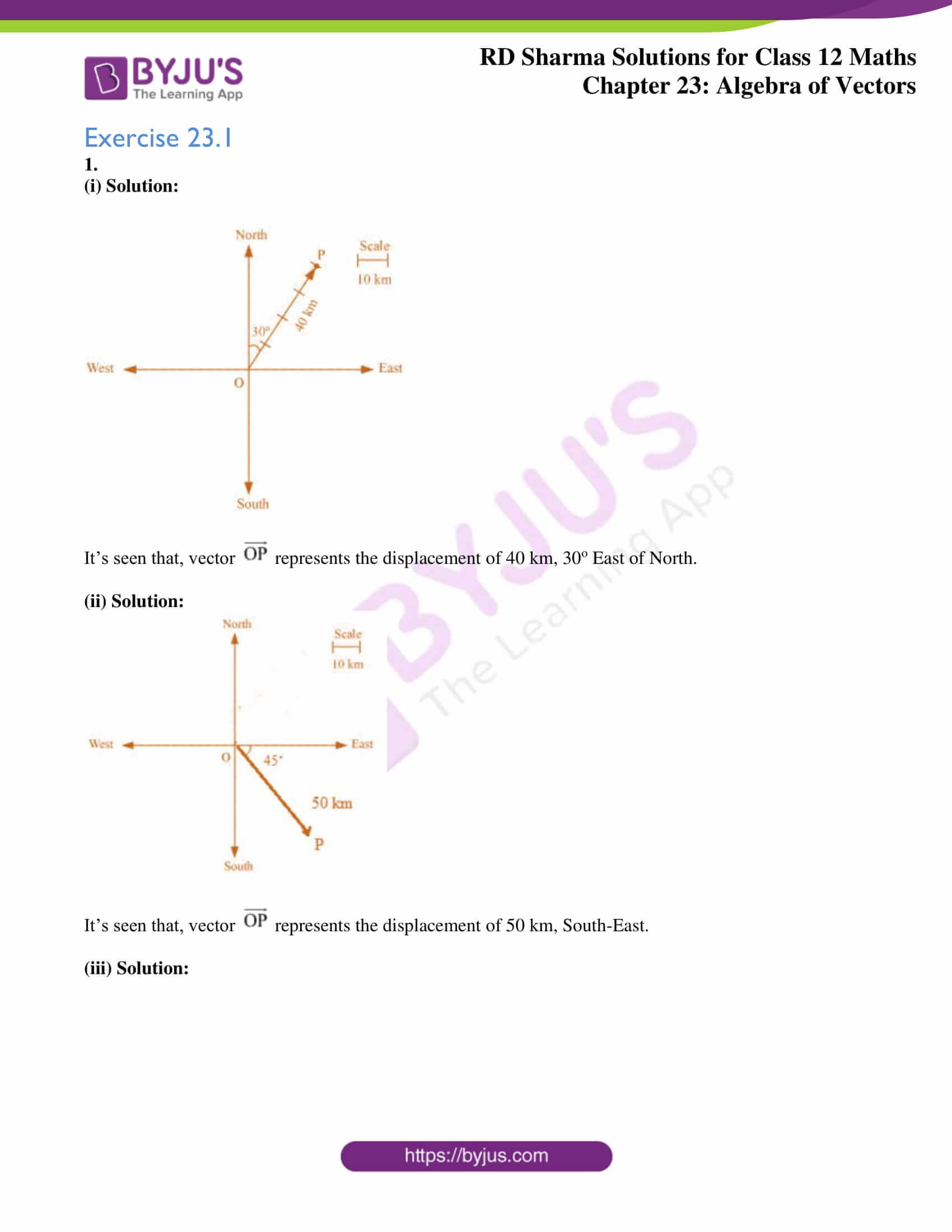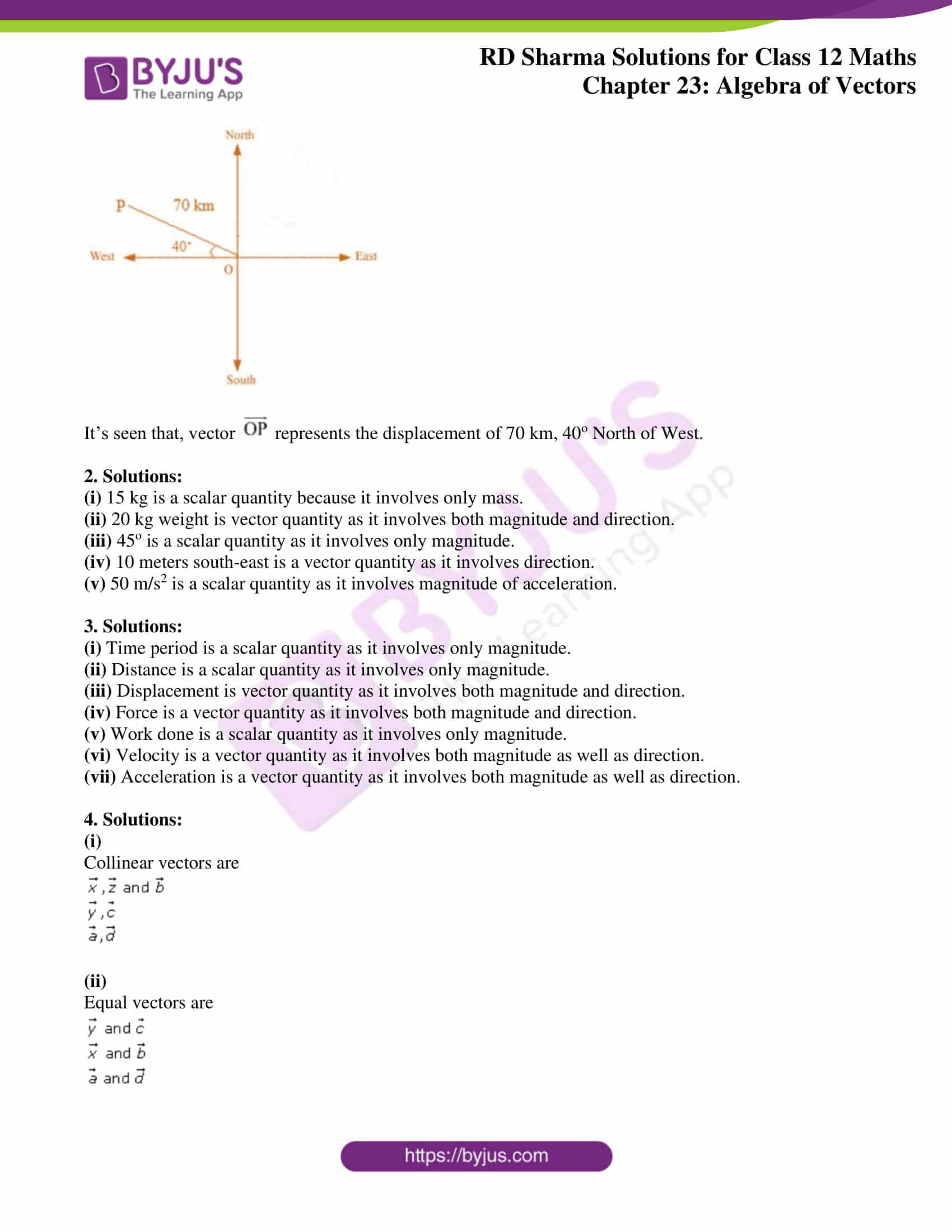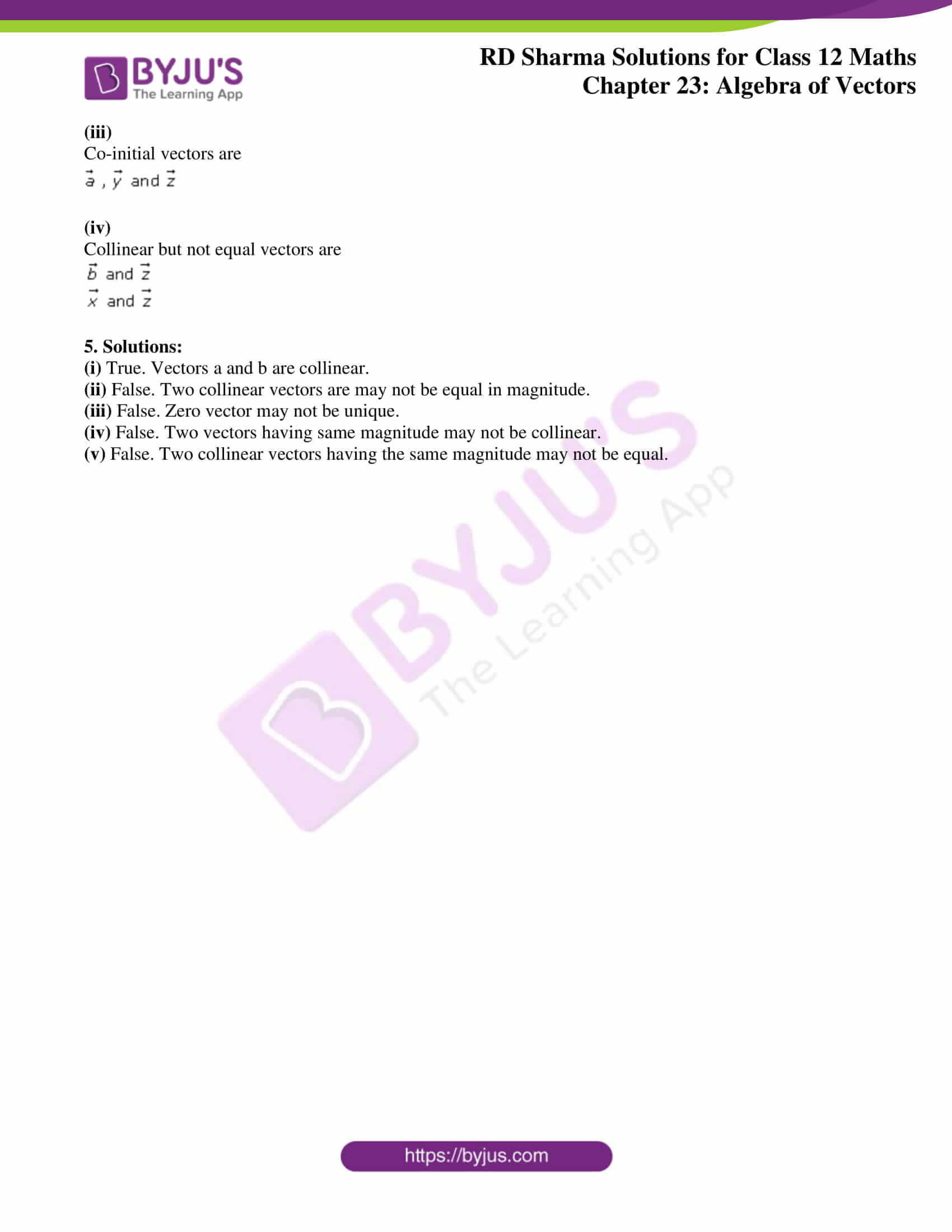### Access Answers for Rd Sharma Solution Class 12 Maths Chapter 23 Exercise 1

Exercise 23.1

1.

(i) Solution: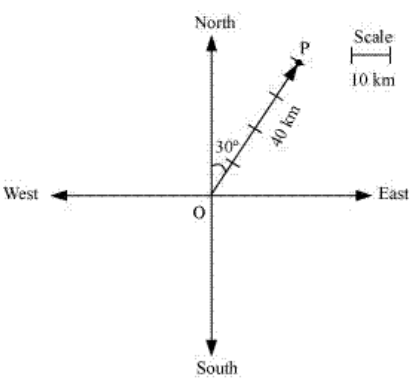It’s seen that, vector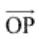represents the displacement of 40 km, 30o East of North.

(ii) Solution: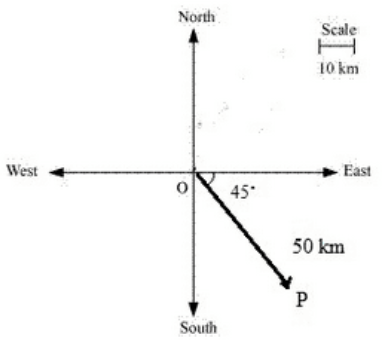It’s seen that, vectorrepresents the displacement of 50 km, South-East.

(iii) Solution: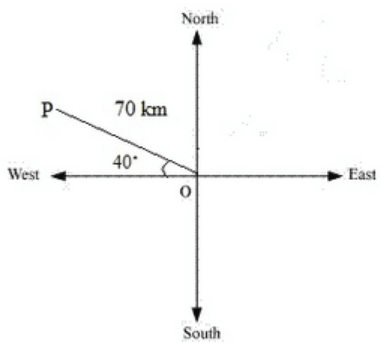It’s seen that, vectorrepresents the displacement of 70 km, 40o North of West.

2. Solutions:

(i) 15 kg is a scalar quantity because it involves only mass.

(ii) 20 kg weight is vector quantity as it involves both magnitude and direction.

(iii) 45o is a scalar quantity as it involves only magnitude.

(iv) 10 meters south-east is a vector quantity as it involves direction.

(v) 50 m/s2 is a scalar quantity as it involves magnitude of acceleration.

3. Solutions:

(i) Time period is a scalar quantity as it involves only magnitude.

(ii) Distance is a scalar quantity as it involves only magnitude.

(iii) Displacement is vector quantity as it involves both magnitude and direction.

(iv) Force is a vector quantity as it involves both magnitude and direction.

(v) Work done is a scalar quantity as it involves only magnitude.

(vi) Velocity is a vector quantity as it involves both magnitude as well as direction.

(vii) Acceleration is a vector quantity as it involves both magnitude as well as direction.

4. Solutions:

(i)

Collinear vectors are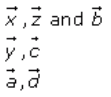(ii)

Equal vectors are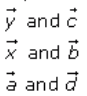(iii)

Co-initial vectors are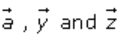(iv)

Collinear but not equal vectors are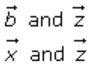5. Solutions:

(i) True. Vectors a and b are collinear.

(ii) False. Two collinear vectors are may not be equal in magnitude.

(iii) False. Zero vector may not be unique.

(iv) False. Two vectors having same magnitude may not be collinear.

(v) False. Two collinear vectors having the same magnitude may not be equal.